## General Question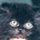# Is it possible to create this image with one continuous line and without going through the same line twice?

Asked by sunssi (120) May 28th, 2011

http://img64.imageshack.us/i/imageyeg.jpg/

Also how can I easily show if any variation of this type of question is true or not? Or is it a NP hard problem?

Observing members: 0Composing members: 0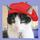You can do trial and error for the next 24 hrs. or find the mathematical proof. It should be on google, as this is a common type of puzzle.

gailcalled (54639)“Great Answer” (2) Flag as…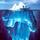It’s impossible. This is covered by Euler’s Theorem in graph theory. It goes back to the 1700s. (Here and here). A graph (consisting of vertex points connected by line segments) cannot be traced without lifting the pencil if there are more than two odd vertices. A vertex is odd or even depending on how many line segments connect to it. In your graph, all three bottom vertices are odd (3, 5, 3). That makes it impossible.

It makes intuitive sense: An even vertex can be entered and exited again an integral number of times. An odd, vertex, however, must represent either the start or finish of thbe path. Where does that leave the 3rd vertex?

gasman (11315)“Great Answer” (19) Flag as…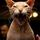No, it’s not possible. I just worked on it for about 20 minutes. You can do the first house very easily, but not with the second one attatched.

WillWorkForChocolate (23160)“Great Answer” (1) Flag as…@WillWorkForChocolate: It’s quicker to do the math apparently.

gailcalled (54639)“Great Answer” (0) Flag as…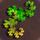It is not possible and here is why:

If the graph is traceable, all vertices must have an even number of connections from it, except for possibly two of them. This is because you must both arrive and leave at every point except for the start and the end. You may visit each point any number of times, but since you must both arrive and leave (2 connections) each time you visit a vertex, the total number of connections for a transversed vertex is

2*(# of visits) = # of connections, an even number

If the start and the end are on different vertices, those two would have an odd number. This is a quick method of finding the start and finish vertices, by locating the two vertices with an odd number of connections. If the graph has more than two vertices with an odd number, then is not traceable. If one vertex is the start and a second is the end, then the others must be transversed and the above formula applies to the number of connections. For the formula to give the correct number of connections for those other odd vertices, we must have visited them a fractional number of times, which is not possible.

In the example, we have four vertices with an odd number of connections. These four points have 3, 3, 7, and 5 connections. If the two 3’s are the start and end, then we must have visited the vertex with 7 connections 3.5 times and the vertex with 5 connections 2.5 times, a logical impossibility.

PhiNotPi (12680)“Great Answer” (1) Flag as…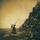Here’s a more fluffy answer for this specific drawing:

Three of the vertexes are odd. (the one connecting the two roofs, and the two outside bottom corners of the drawing)

So even if you chose one of them as your start point, and the other as your end point, you’re still gonna have that third one to deal with. And since it has an odd number of lines connecting to it, you will not be able to draw all those lines and still continue to your chosen end point.

adr (434)“Great Answer” (0) Flag as…or